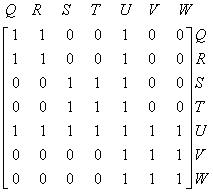Java/JBLAS: Calculating eigenvector centrality of an adjacency matrix

I recently came across a very interesting post by Kieran Healy where he runs through a bunch of graph algorithms to see whether he can detect the most influential people behind the American Revolution based on their membership of various organisations.

The first algorithm he looked at was betweenness centrality which I've looked at previously and is used to determine the load and importance of a node in a graph.

This algorithm would assign a high score to nodes which have a lot of nodes connected to them even if those nodes aren't necessarily influential nodes in the graph.

If we want to take the influence of the other nodes into account then we can use an algorithm called eigenvector centrality.

Eigenvector centrality is a measure of the influence of a node in a network. It assigns relative scores to all nodes in the network based on the concept that connections to high-scoring nodes contribute more to the score of the node in question than equal connections to low-scoring nodes. Google's PageRank is a variant of the Eigenvector centrality measure.

Both PageRank and Eigenvector centrality give us a probability which describes how often we'd end up visiting each node on a random walk around the graph.

As far as I can tell there are a couple of differences between PageRank and Eigenvector centrality (but I'm happy to be corrected as I'm still learning this stuff):

1. PageRank introduces a 'dampening factor' to simulate the idea that some percentage of the time we might decide not to follow any of a node's relationships but instead pick a random node in the graph.
2. PageRank makes sure that the elements in each column of the adjacency matrix add up to one. Therefore, if our node had a relationship to every other one in the graph then each would only contribute a value of 1/n rather than 1.

In this instance since Healy wanted to analyse the influence of people rather than web pages eigenvector centrality makes more sense.

Over the past few days I've been trying to understand this topic area a bit better and found the following resources useful:

I calculated a few made up matrices by hand but found it became too difficult after a 3x3 matrix so I wanted to find a Java based library which I could use instead.These were the ones that I came across:

• JBLAS - Linear Algebra for Java
• JAMA - A Java Matrix Package
• Colt - Advanced Computing for Science
• Commons Math - The Apache Commons Mathematics Library
• la4j - Linear Algebra for Java
• MTJ - Matrix Toolkits Java

I'd heard of JBLAS before so I thought I'd give that a try on one of the adjacency matrices described in Murphy Waggoner's post about the Gould Index and see if I got the same eigenvector centrality values.

The first step was to define the matrix which can be represented as an array of arrays:

``````
DoubleMatrix matrix = new DoubleMatrix(new double[][] {
{1,1,0,0,1,0,0},
{1,1,0,0,1,0,0},
{0,0,1,1,1,0,0},
{0,0,1,1,1,0,0},
{1,1,1,1,1,1,1},
{0,0,0,0,1,1,1},
{0,0,0,0,1,1,1},
});
``````

Our next stop is to work out the eigenvalues which we can do using the following function:

``````
ComplexDoubleMatrix eigenvalues = Eigen.eigenvalues(matrix);
for (ComplexDouble eigenvalue : eigenvalues.toArray()) {
System.out.print(String.format("%.2f ", eigenvalue.abs()));
}
``````
``````
4.00 2.00 0.00 1.00 2.00 0.00 0.00
``````

We want to get the corresponding eigenvector for the eigenvalue of 4 and as far as I can tell the Eigen#eigenvectors function returns its values in the same order as the Eigen#eigenvalues function so I wrote the following code to work out the principal eigenvector :

``````
List<Double> principalEigenvector = getPrincipalEigenvector(matrix);
System.out.println("principalEigenvector = " + principalEigenvector);

private static List<Double> getPrincipalEigenvector(DoubleMatrix matrix) {
int maxIndex = getMaxIndex(matrix);
ComplexDoubleMatrix eigenVectors = Eigen.eigenvectors(matrix);
return getEigenVector(eigenVectors, maxIndex);
}

private static int getMaxIndex(DoubleMatrix matrix) {
ComplexDouble[] doubleMatrix = Eigen.eigenvalues(matrix).toArray();
int maxIndex = 0;
for (int i = 0; i < doubleMatrix.length; i++){
double newnumber = doubleMatrix[i].abs();
if ((newnumber > doubleMatrix[maxIndex].abs())){
maxIndex = i;
}
}
return maxIndex;
}

private static List<Double> getEigenVector(ComplexDoubleMatrix eigenvector, int columnId) {
ComplexDoubleMatrix column = eigenvector.getColumn(columnId);

List<Double> values = new ArrayList<Double>();
for (ComplexDouble value : column.toArray()) {
}
return values;
}
``````

In getMaxIndex we work out which index in the array the largest eigenvalue belongs to so that we can look it up in the array we get from Eigen#eigenvectors. According to the documentation the eigenvectors are stored in the first matrix we get back which is why we choose that on the second line of getPrincipalEigenvector.

This is the output we get from running that:

``````
principalEigenvector = [0.3162277660168381, 0.3162277660168376, 0.316227766016838, 0.316227766016838, 0.6324555320336759, 0.316227766016838, 0.316227766016838]
``````

Finally we normalise the values so that they all add together to equal 1 which means our result will tell the % of time that a random walk would take you to this node:

``````
System.out.println("normalisedPrincipalEigenvector = " + normalised(principalEigenvector));

private static List<Double> normalised(List<Double> principalEigenvector) {
double total = sum(principalEigenvector);
List<Double> normalisedValues = new ArrayList<Double>();
for (Double aDouble : principalEigenvector) {
}
return normalisedValues;
}

private static double sum(List<Double> principalEigenvector) {
double total = 0;
for (Double aDouble : principalEigenvector) {
``````